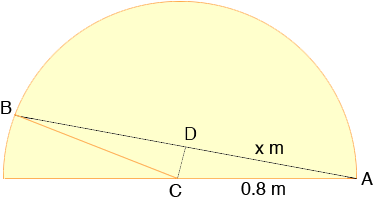Quandaries and Queries Hi My question is how do you determine the length of a chord when given the diameter of the circle (1.6m) and that the angle = 7pi/8 Please help Many thanks Sue elementary (KS 3) I am the mum of a student who is struggling and would like to help them but before I can I need to know how it is worked out. Hi Sue,In the diagram the measure of the angle BCA is 7/8and CD bisects this angle so the measure of the angle DCA is 7/16. The length of AD is x m and D is the midpoint of AB so the length of the chord is 2x m. (One thing I can see from the diagram is that the chord is almost as long as the diameter so the length of the chord will be almost 1.6 m.) The triangle DCA is a right triangle and hence the sine of the angle DCA is x/0.8. Thus sin( 7/16) =  x/0.8 so x = 0.8sin( 7/16) Using my calculator to find the value of the sine I got x = 0.7846 m and thus the length of the chord is 1.57 m. Penny